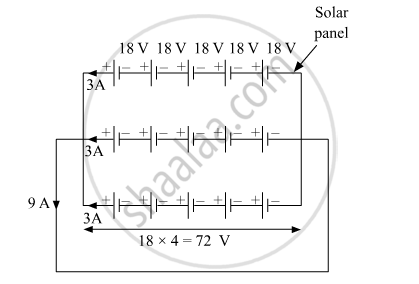# Draw Neat and Labelled Diagram. One Solar Panel Produces a Potential Difference of 18 V and Current of 3 A. Discribe How You Can Obtain a Potential Difference of 72 Volts and Current of - Science and Technology 2

Draw neat and labelled diagram.

One solar panel produces a potential difference of 18 V and current of 3 A. Discribe how you can obtain a potential difference of 72 Volts and current of 9 A with a solar array using solar panels. You can use sign of a battery for a solar panel.

#### Solution

We can obtain a potential difference of 72 V by forming a string of 5 solar panels in series. Now, to obtain current of 9 A, connect 3 such identical strings in parallel. The same has been shown below.Concept: Solar Energy
Is there an error in this question or solution?